Скачать презентацию Chapter 5 Simple Modelling Where we examine various

07764a13f69d08c67a13b00255fc5be8.ppt

• Количество слайдов: 27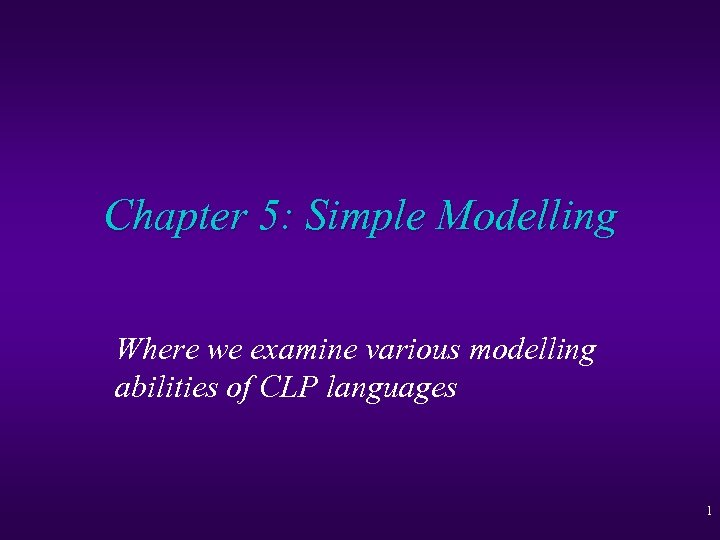Chapter 5: Simple Modelling Where we examine various modelling abilities of CLP languages 1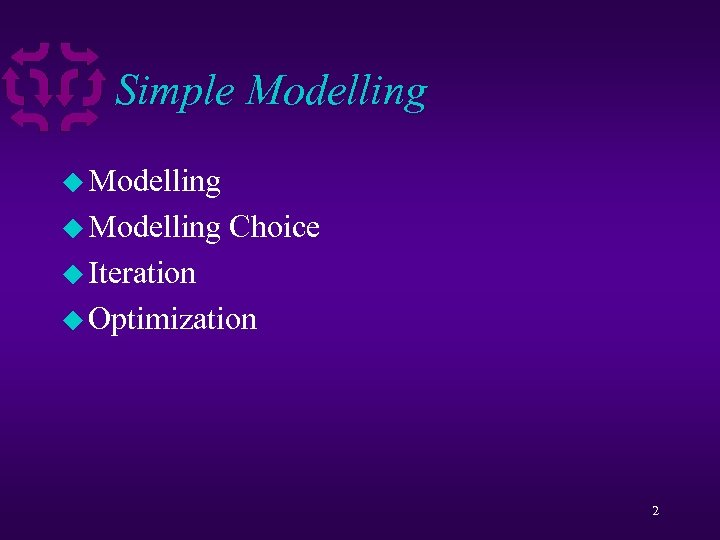Simple Modelling u Modelling Choice u Iteration u Optimization 2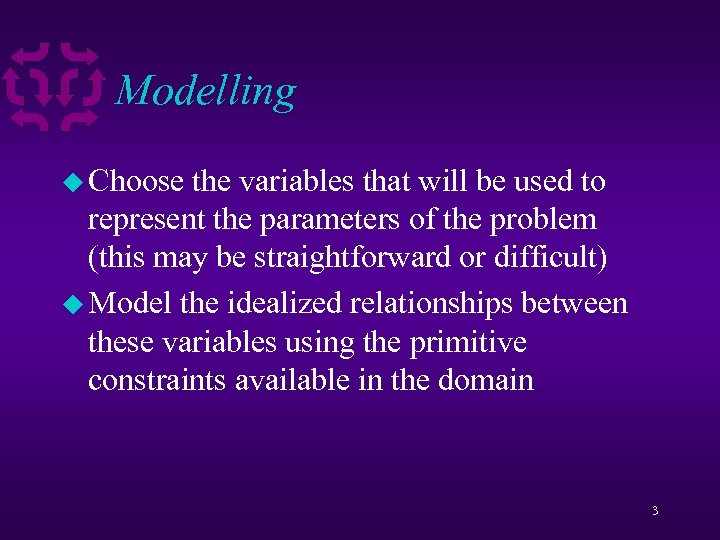Modelling u Choose the variables that will be used to represent the parameters of the problem (this may be straightforward or difficult) u Model the idealized relationships between these variables using the primitive constraints available in the domain 3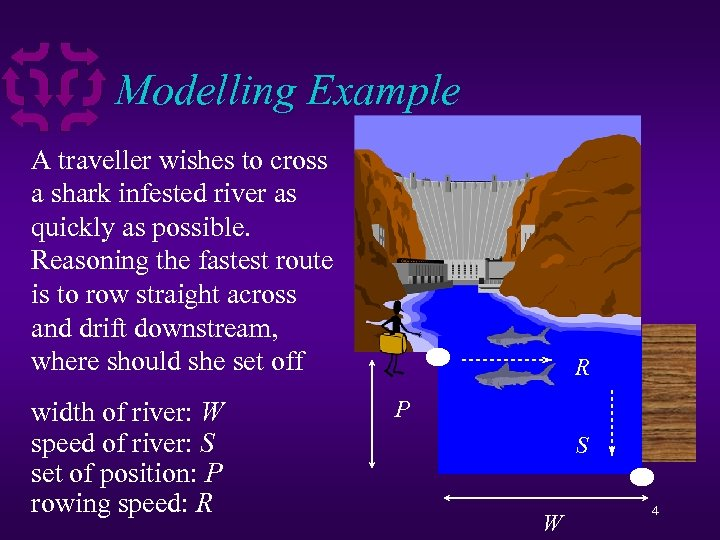Modelling Example A traveller wishes to cross a shark infested river as quickly as possible. Reasoning the fastest route is to row straight across and drift downstream, where should she set off width of river: W speed of river: S set of position: P rowing speed: R R P S W 4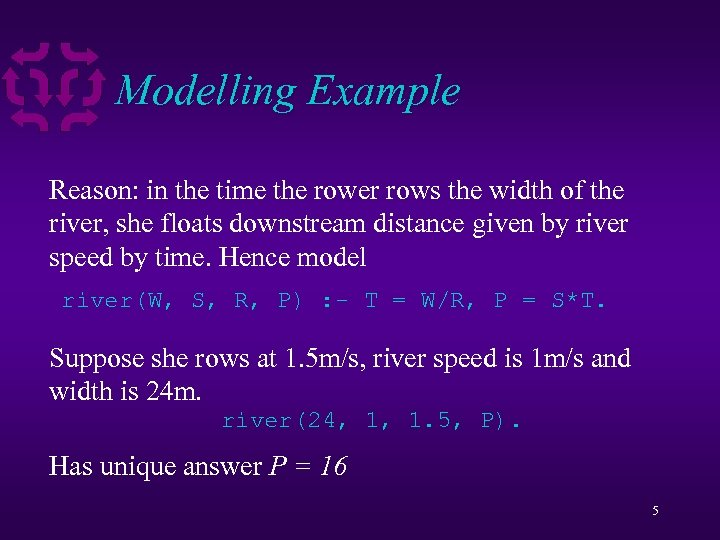Modelling Example Reason: in the time the rower rows the width of the river, she floats downstream distance given by river speed by time. Hence model river(W, S, R, P) : - T = W/R, P = S*T. Suppose she rows at 1. 5 m/s, river speed is 1 m/s and width is 24 m. river(24, 1, 1. 5, P). Has unique answer P = 16 5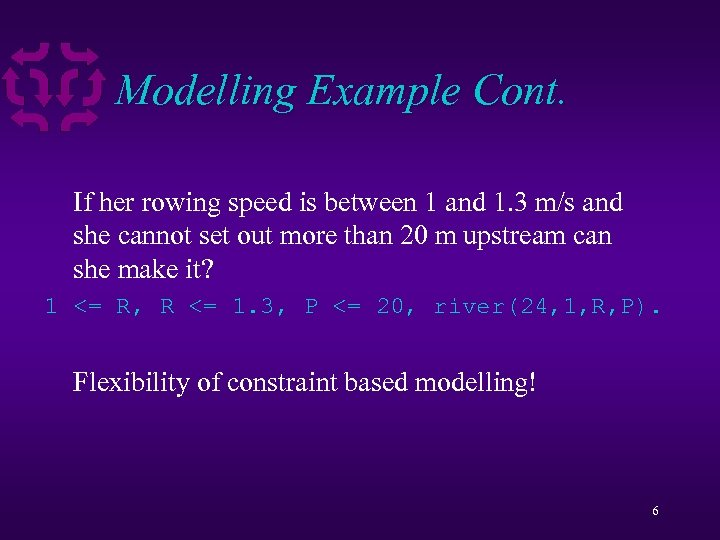Modelling Example Cont. If her rowing speed is between 1 and 1. 3 m/s and she cannot set out more than 20 m upstream can she make it? 1 <= R, R <= 1. 3, P <= 20, river(24, 1, R, P). Flexibility of constraint based modelling! 6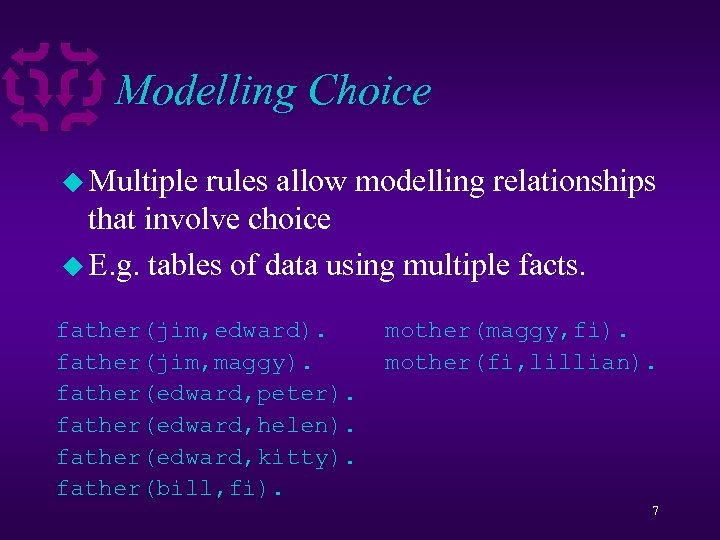Modelling Choice u Multiple rules allow modelling relationships that involve choice u E. g. tables of data using multiple facts. father(jim, edward). father(jim, maggy). father(edward, peter). father(edward, helen). father(edward, kitty). father(bill, fi). mother(maggy, fi). mother(fi, lillian). 7Choice Examples The goal father(edward, X) finds children of Edward. Answers: The goal mother(X, fi)finds the mother of Fi. Answers: 8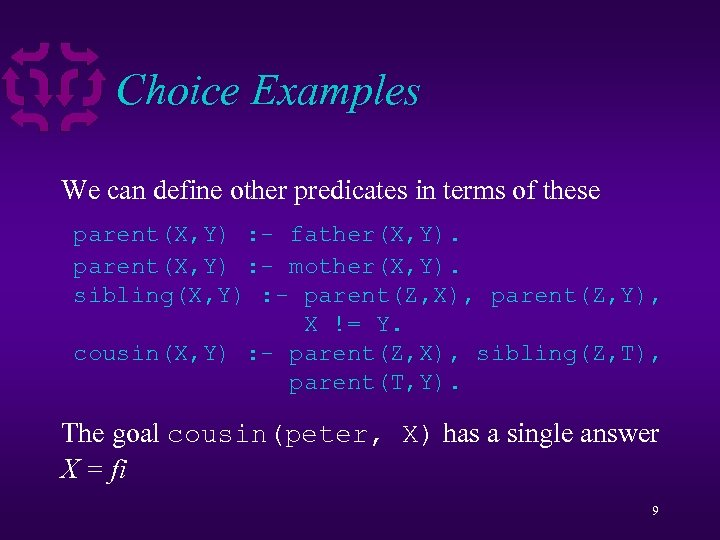Choice Examples We can define other predicates in terms of these parent(X, Y) : - father(X, Y). parent(X, Y) : - mother(X, Y). sibling(X, Y) : - parent(Z, X), parent(Z, Y), X != Y. cousin(X, Y) : - parent(Z, X), sibling(Z, T), parent(T, Y). The goal cousin(peter, X) has a single answer X = fi 9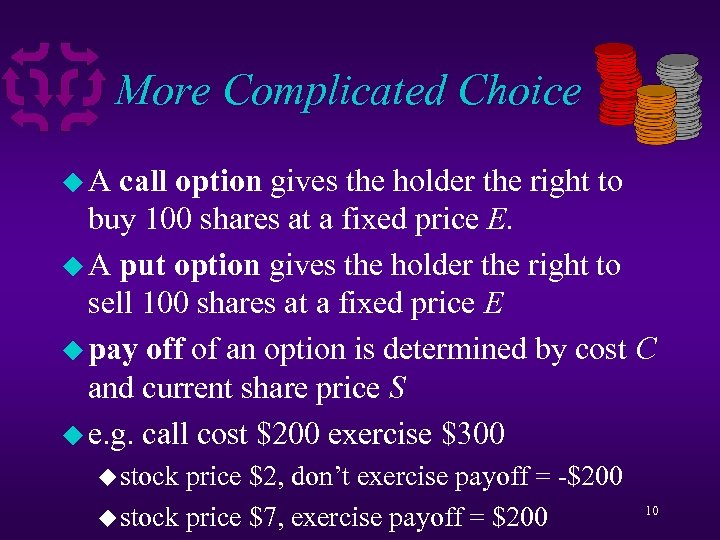More Complicated Choice u. A call option gives the holder the right to buy 100 shares at a fixed price E. u A put option gives the holder the right to sell 100 shares at a fixed price E u pay off of an option is determined by cost C and current share price S u e. g. call cost \$200 exercise \$300 u stock price \$2, don’t exercise payoff = -\$200 u stock price \$7, exercise payoff = \$200 10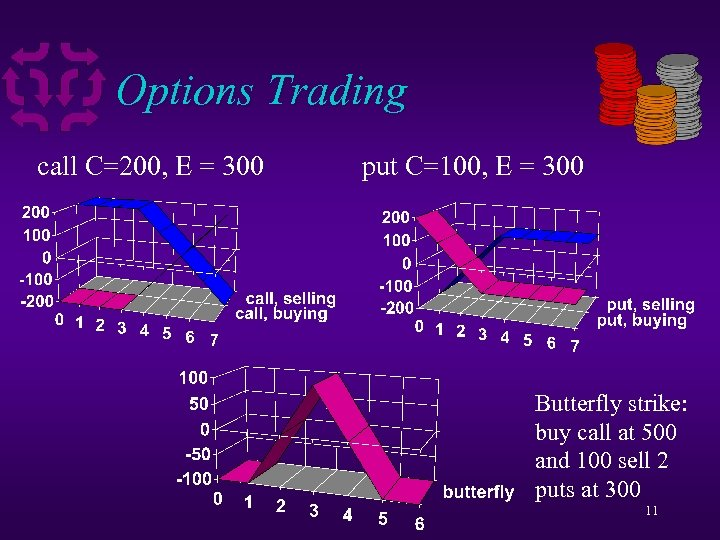Options Trading call C=200, E = 300 put C=100, E = 300 Butterfly strike: buy call at 500 and 100 sell 2 puts at 300 11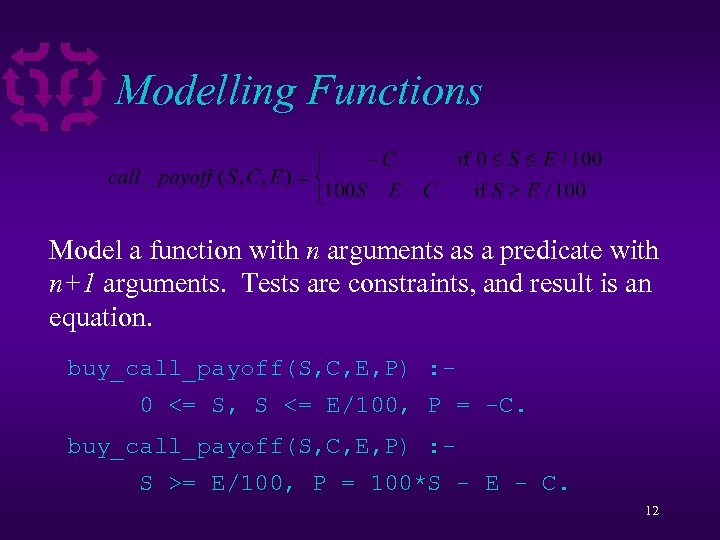Modelling Functions Model a function with n arguments as a predicate with n+1 arguments. Tests are constraints, and result is an equation. buy_call_payoff(S, C, E, P) : 0 <= S, S <= E/100, P = -C. buy_call_payoff(S, C, E, P) : S >= E/100, P = 100*S - E - C. 12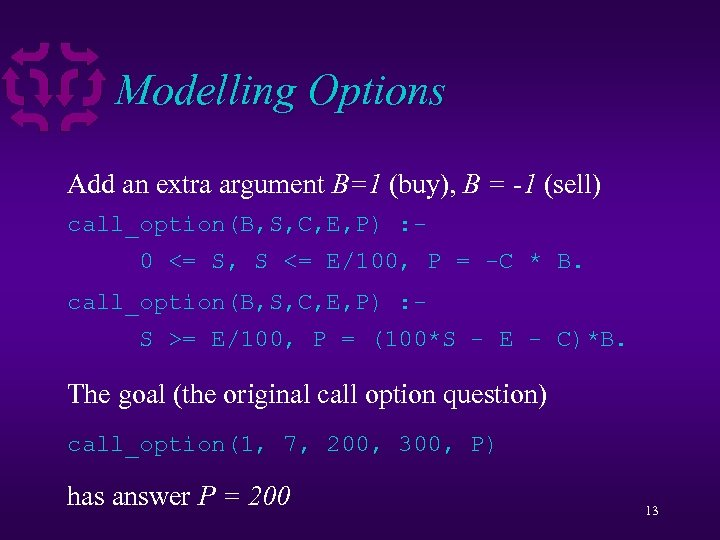Modelling Options Add an extra argument B=1 (buy), B = -1 (sell) call_option(B, S, C, E, P) : 0 <= S, S <= E/100, P = -C * B. call_option(B, S, C, E, P) : S >= E/100, P = (100*S - E - C)*B. The goal (the original call option question) call_option(1, 7, 200, 300, P) has answer P = 200 13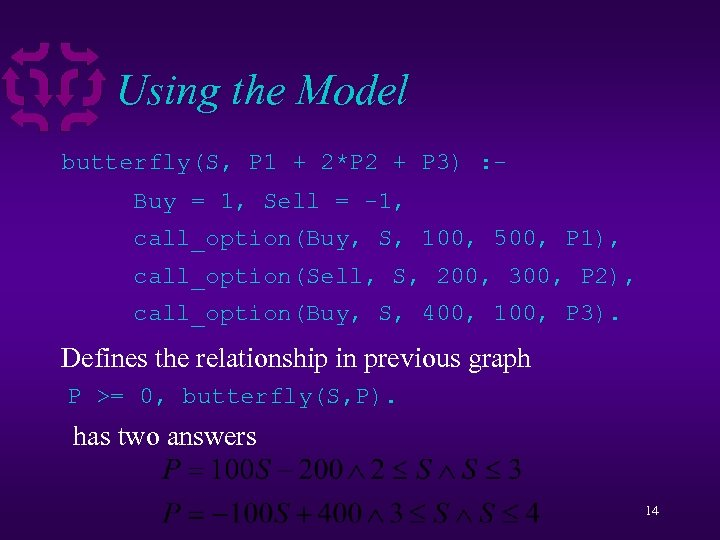Using the Model butterfly(S, P 1 + 2*P 2 + P 3) : Buy = 1, Sell = -1, call_option(Buy, S, 100, 500, P 1), call_option(Sell, S, 200, 300, P 2), call_option(Buy, S, 400, 100, P 3). Defines the relationship in previous graph P >= 0, butterfly(S, P). has two answers 14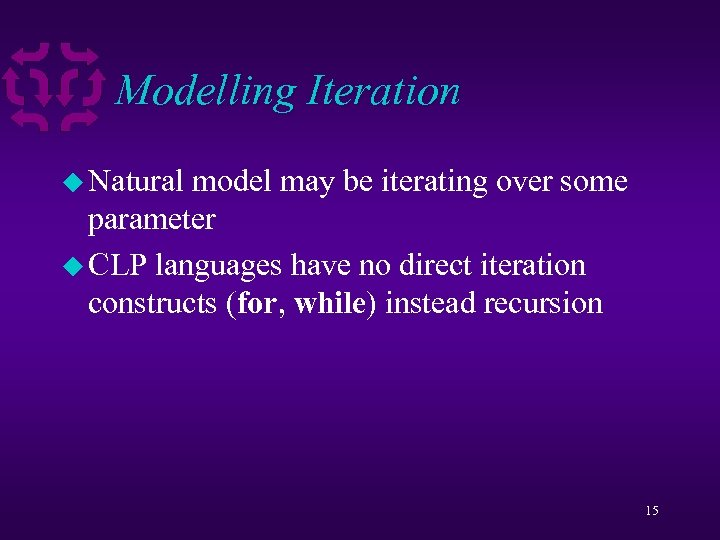Modelling Iteration u Natural model may be iterating over some parameter u CLP languages have no direct iteration constructs (for, while) instead recursion 15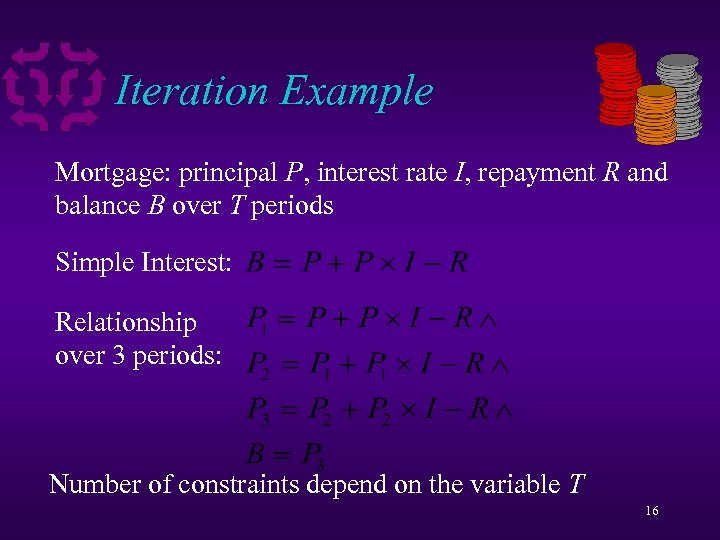Iteration Example Mortgage: principal P, interest rate I, repayment R and balance B over T periods Simple Interest: Relationship over 3 periods: Number of constraints depend on the variable T 16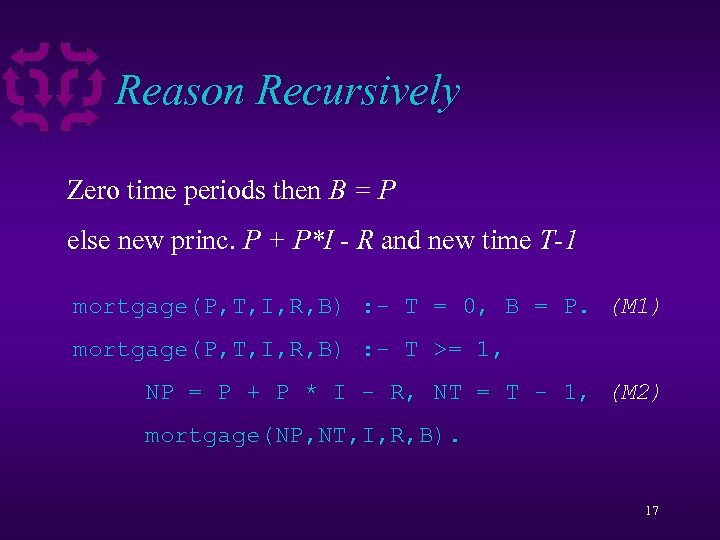Reason Recursively Zero time periods then B = P else new princ. P + P*I - R and new time T-1 mortgage(P, T, I, R, B) : - T = 0, B = P. (M 1) mortgage(P, T, I, R, B) : - T >= 1, NP = P + P * I - R, NT = T - 1, (M 2) mortgage(NP, NT, I, R, B). 17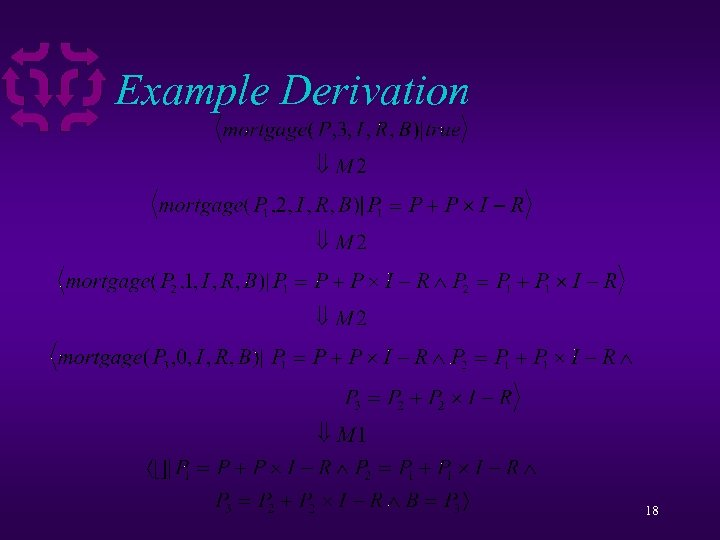Example Derivation 18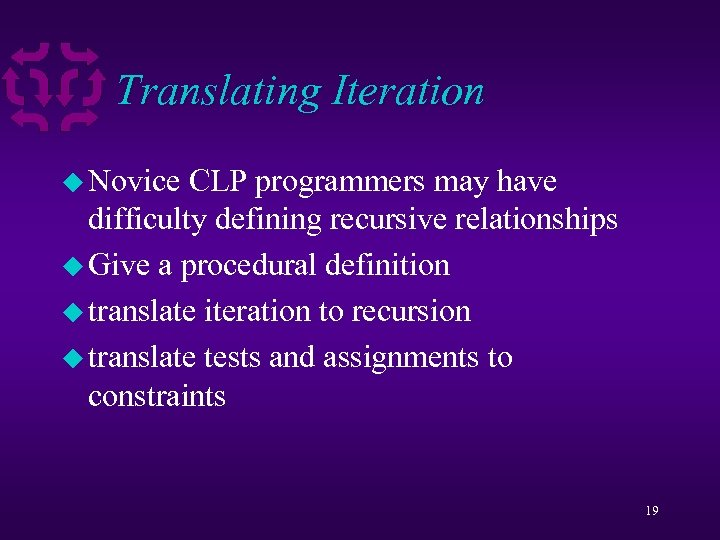Translating Iteration u Novice CLP programmers may have difficulty defining recursive relationships u Give a procedural definition u translate iteration to recursion u translate tests and assignments to constraints 19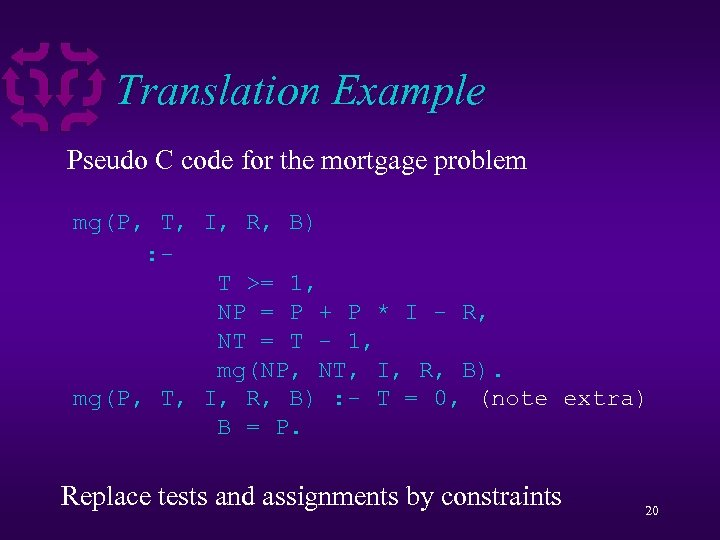Translation Example Pseudo C code for the mortgage problem float mg 1(float P, int I, float *B) float mg 2(float B) int I, float R) mg 4(float I, R, P, mg(P, mg 3(float I, float R, float R) T, P, int { { { : while (T >= 1) { if (T T >= 1, if (T >= 1) { P ==PP++PP**II--R; NP = P + P * I - R; NP R, T ==TT--1; NT = T - 1; NT 1, } return mg 2(P, T, I, I, R) B) return mg 3(NP, R, B). R, return mg 4(NP, NT, I, mg(NP, NT, I, NT, R) return P; else mg(P, T, I, R, B) : - T = 0, (note extra) } return P; *B = P; } } } Make each function with a procedure answer by ref. Remove thevariable only take recursion the and loop using by value Replace testswhileassignmentsone constraints 20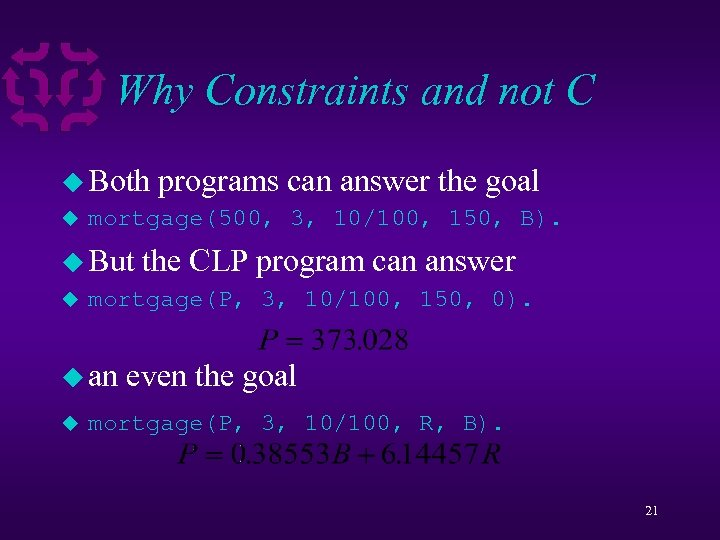Why Constraints and not C u Both programs can answer the goal u mortgage(500, 3, 10/100, 150, B). u But the CLP program can answer u mortgage(P, 3, 10/100, 150, 0). u an u even the goal mortgage(P, 3, 10/100, R, B). 21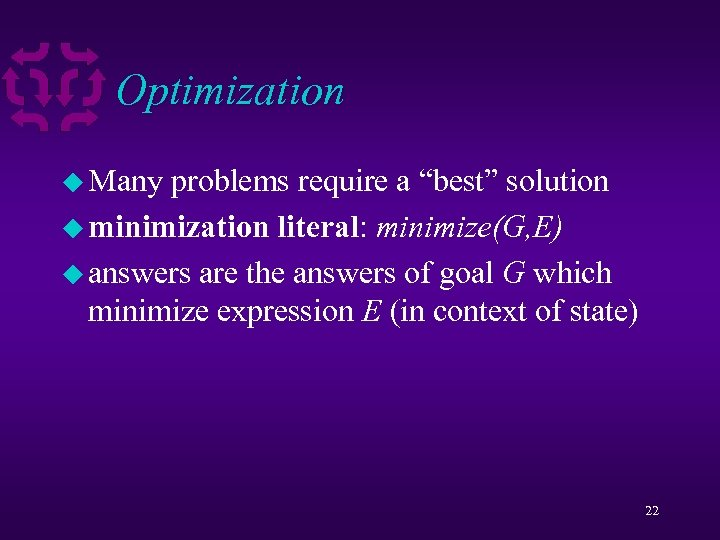Optimization u Many problems require a “best” solution u minimization literal: minimize(G, E) u answers are the answers of goal G which minimize expression E (in context of state) 22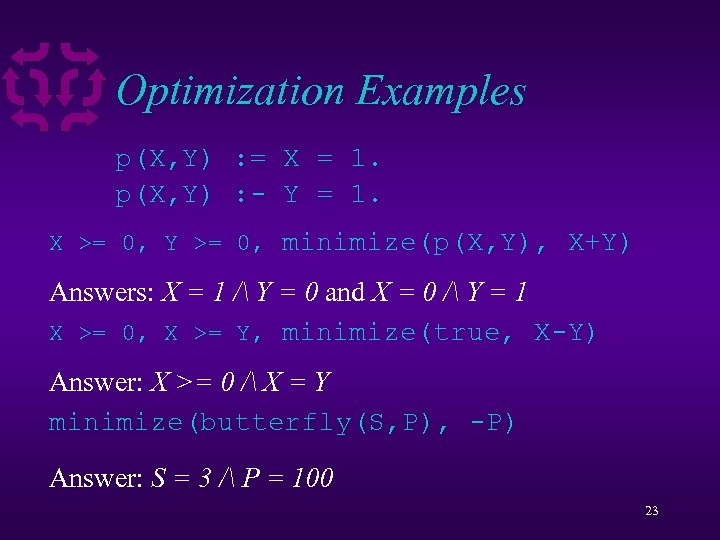Optimization Examples p(X, Y) : = X = 1. p(X, Y) : - Y = 1. X >= 0, Y >= 0, minimize(p(X, Y), X+Y) Answers: X = 1 / Y = 0 and X = 0 / Y = 1 X >= 0, X >= Y, minimize(true, X-Y) Answer: X >= 0 / X = Y minimize(butterfly(S, P), -P) Answer: S = 3 / P = 100 23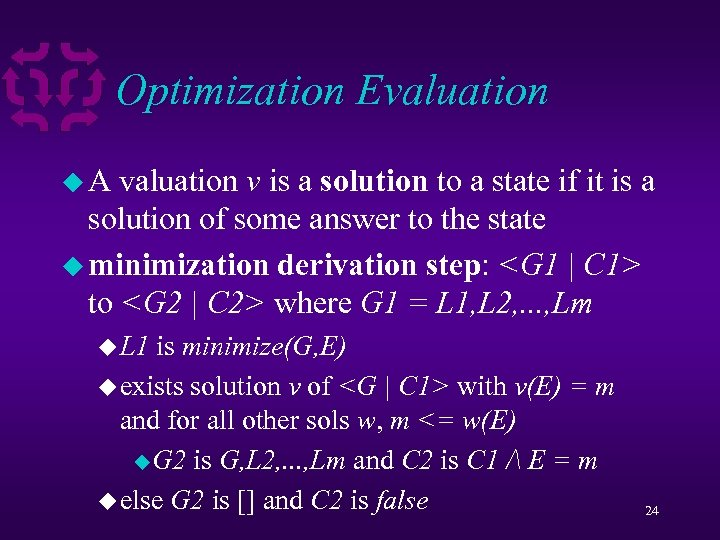Optimization Evaluation u. A valuation v is a solution to a state if it is a solution of some answer to the state u minimization derivation step: to where G 1 = L 1, L 2, . . . , Lm u L 1 is minimize(G, E) u exists solution v of with v(E) = m and for all other sols w, m <= w(E) u. G 2 is G, L 2, . . . , Lm and C 2 is C 1 / E = m u else G 2 is [] and C 2 is false 24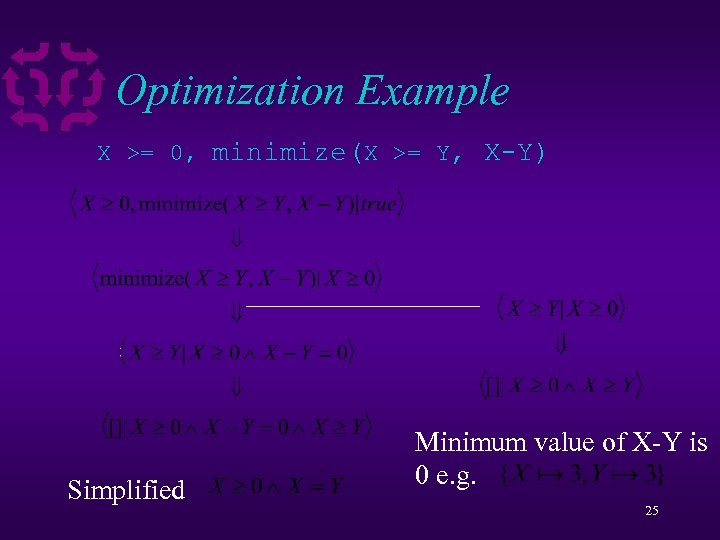Optimization Example X >= 0, minimize(X >= Y, X-Y) Simplified Minimum value of X-Y is 0 e. g. 25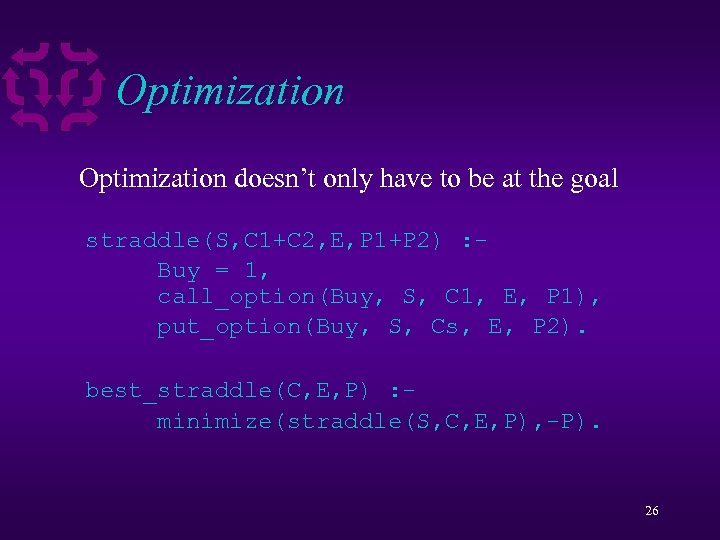Optimization doesn’t only have to be at the goal straddle(S, C 1+C 2, E, P 1+P 2) : Buy = 1, call_option(Buy, S, C 1, E, P 1), put_option(Buy, S, Cs, E, P 2). best_straddle(C, E, P) : minimize(straddle(S, C, E, P), -P). 26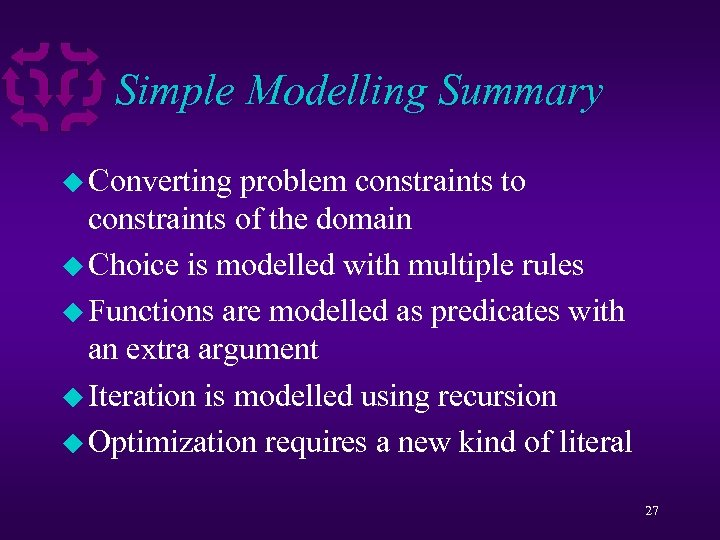Simple Modelling Summary u Converting problem constraints to constraints of the domain u Choice is modelled with multiple rules u Functions are modelled as predicates with an extra argument u Iteration is modelled using recursion u Optimization requires a new kind of literal 27